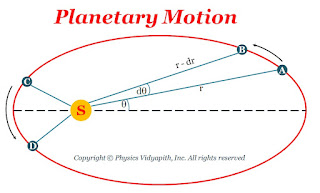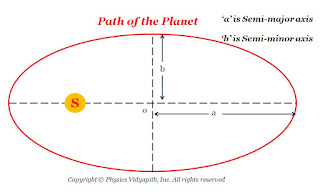### Kepler's Laws of Planetary Motion

Kepler's Laws →

Kepler's found important uniformity in the motion of the planets. These uniformity are known as "Kepler's Laws of planetary motion". There are basically three laws →
1. First law (Law of orbits)

2. Second Law (Law of Areal Speed)

3. Third Law (Law of Periods)

First law (Law of orbits) →
All the planets move around the sun in elliptical path and the sun is at the one foci of the ellipse.

Second Law (Law of Areal Speed) →
A line joining the any planet to sun sweeps out equal areas in equal interval of of times. i.e The areal speed of the planets remains constant.

According to the second law, When the planet is farthest from the sun, then its speed is maximum, and when it is nearest the sun, then its speed is maximum.Planetary Motion
From the above figure, A planet is moving around the sun from $A$ to $B$ in a given time interval, and from $C$ to $D$ in the same time interval, then according to the law of areal speed, the areas $ASB$ and $CSD$ will be equal with respect to the same time-interval

Proof →

Let us consider, A planet moves the angle $d\theta$ from $A$ to $B$ in the infinitesimal interval of time $dt$, then the area swept out by radial line $SA$ is

$dA$= Area of the curved $\Delta$ $SAB$

$dA \approx \frac{1}{2} \left( AB \times SA \right)$

$dA \approx \frac{1}{2} \left( r \: d\theta \times r \right)$

$dA \approx \frac{1}{2} \left( r^{2} \: d\theta \right)$

Thus, the instantaneous areal speed of the planets is

$\frac{dA}{dt}=\frac{1}{2} r^{2} \frac{d \theta}{dt}$

$\frac{dA}{dt}=\frac{1}{2} r^{2} \omega \qquad(1)$

Where $\omega$ → The angular speed of the planet.

We know that

$J=I \: \omega$

Where $I$ is the instantaneous moment of inertia of the planet about the Sun $S$.

$J=m \: r^{2} \: \omega \qquad(2)$

Where $m$ is the mass of the planet. Now substitute the value of $r^{2} \: \omega$ from equation $(2)$ in equation $(1)$.

 $\frac{dA}{dt}=\frac{J}{2m} \left( Constant \right)$

From the above equation, The areal speed $\frac{dA}{dt}$ of the planet is constant which is Kepler's Second Law. The above equation shows that the angular momentum of the planet is constant. i.e The angular momentum of any planet is conserved.

Third Law (Law of Periods) →
The square of the time period of one complete revolution of any planet around the sun is directly proportional to the cube of the semi-major axis of its elliptical orbit.Path of the Planet

Proof →

Let $a$ and $b$ be the semi-major and semi-minor axes of the ellipse, then the area of the ellipse will be $\pi ab$. Let $T$ is the period of one complete revolution of any planet, then

$T=\frac{Area \: of \: the \: ellipse}{Areal \: Speed}$

$T=\frac{\pi a b}{\frac{J}{2m}}$

$T=\frac{2\pi a b m}{J}$

$T^{2}=\frac{4 \pi^{2} a^{2} b^{2} m^{2}}{J^{2}}$

Let $l$ is the semi latus rectum of the elliptical orbit i.e $l=\frac{b^{2}}{a}$. Then we get the above equation which can be written as

$T^{2}=\frac{4 \pi^{2} m^{2} a^{3} l }{J^{2}}$

 $T^{2} \propto a^{3}$

Where $\frac{4 \pi^{2} m^{2} l }{J^{2}}$ is constant.

From the above equation, It is clear that the larger the distance of a planet from the sun, the large will be its period of revolution around the sun. The period of revolution of the nearest planet of the sun i.e. Mercury is $88$ days, while that of the faraway planet from the sun i.e. Pluto is $248$ years.